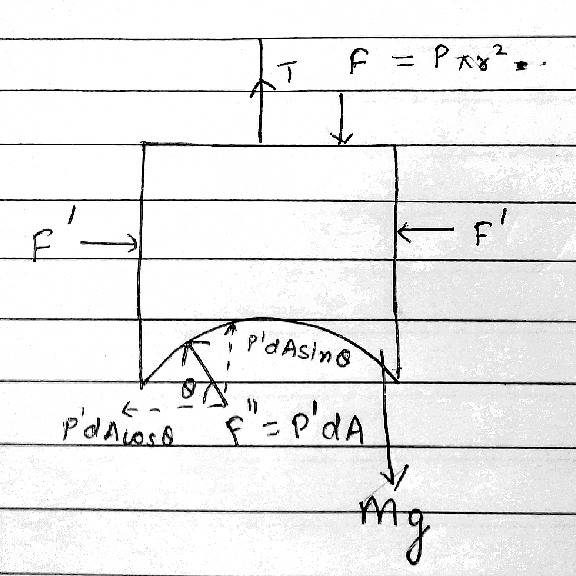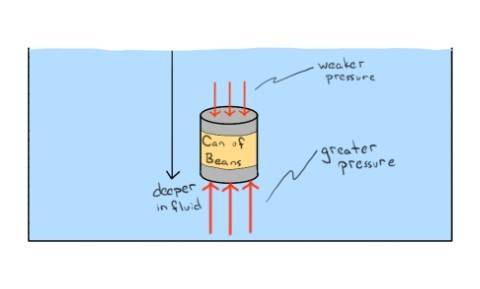# Buoyant force on a submerged body

## Homework Statement

A hemispherical portion of radius R is removed from the bottom of a cylinder of radius R. The volume of the remaining cylinder is V and its mass is M. It is suspended by a string in a liquid of density ρ where it stays vertical. The upper surface of the cylinder is at a depth h below the liquid surface. The force on the bottom of the cylinder by the liquid is?## Homework Equations

Force = Pressure x Area

## The Attempt at a Solution

Since the object is in equilibrium, I balanced the 3 forces acting on it.
Weight of the body = Force on the top of the cylinder - Force on the bottom of the cylinder
⇒Mg = ρgh(πR²) - F (ignoring atmospheric pressure)
⇒F = πR²hρg - Mg
But the answer is F = ρg( V + πR²h)
What did I do wrong?

ehild
Homework Helper

## Homework Statement

A hemispherical portion of radius R is removed from the bottom of a cylinder of radius R. The volume of the remaining cylinder is V and its mass is M. It is suspended by a string in a liquid of density ρ where it stays vertical. The upper surface of the cylinder is at a depth h below the liquid surface. The force on the bottom of the cylinder by the liquid is?
View attachment 222955

## Homework Equations

Force = Pressure x Area

## The Attempt at a Solution

Since the object is in equilibrium, I balanced the 3 forces acting on it.
Weight of the body = Force on the top of the cylinder - Force on the bottom of the cylinder
⇒Mg = ρgh(πR²) - F (ignoring atmospheric pressure)
⇒F = πR²hρg - Mg
But the answer is F = ρg( V + πR²h)
What did I do wrong?

There is the force of the string you omitted.

haruspex
Homework Helper
Gold Member
2020 Award
Hint: think about the forces on the three different surfaces and what they must add up to vectorially.

There is the force of the string you omitted.
Then the equation will become
T - Mg = ρgh(πr²) - F
I have 2 variables now. How can I solved this equation?
Can I assume T ≈ Mg because the string remains taut?

haruspex
Homework Helper
Gold Member
2020 Award
Then the equation will become
T - Mg = ρgh(πr²) - F
I have 2 variables now. How can I solved this equation?
Can I assume T ≈ Mg because the string remains taut?
No need to get tangled up with the string. See my hint in post #3 and remember Archimedes.

Hint: think about the forces on the three different surfaces and what they must add up to vectorially.
3 different surfaces of the cylinder?
I tried.Here the forces on the sides cancel each other.
F''cosθ cancel each other.
According to Archimedes, buoyant force= weight of liquid displaced
= Vρg
⇒ρgh(πr²) - F = Vρg
F = ρg(πr²h - V)
Which is still not correct.

#### Attachments

haruspex
Homework Helper
Gold Member
2020 Award
Which is still not correct.
True, but now it is down to a sign error. Think very carefully which force(s) you might have ascribed the wrong sign to in the equation.

True, but now it is down to a sign error. Think very carefully which force(s) you might have ascribed the wrong sign to in the equation.
It must be the force on the upper surface, according to the answer.
But why? The water above the object will exert a downward force on the object. Because the force should be perpendicular to the surface.
The water below the object will exert a force upward for the same reason.

ehild
Homework Helper
It must be the force on the upper surface, according to the answer.
But why? The water above the object will exert a downward force on the object. Because the force should be perpendicular to the surface.
The water below the object will exert a force upward for the same reason.
What is the direction of the buoyant force? Upward or downward?

What is the direction of the buoyant force? Upward or downward?
Upward.
The direction of net buoyant force is upward.I found this image which relates to what I am confused about.
The force on the upper surface (say F1) is downward, but weaker due to lesser height. The force on lower surface (say F2) is stronger and upward.
Thus net force = Buoyant force = F2 - F1
Why can't we apply the same thing here as well?

#### Attachments

haruspex
Homework Helper
Gold Member
2020 Award
Upward.
The direction of net buoyant force is upward.View attachment 222965
I found this image which relates to what I am confused about.
The force on the upper surface (say F1) is downward, but weaker due to lesser height. The force on lower surface (say F2) is stronger and upward.
Thus net force = Buoyant force = F2 - F1
Why can't we apply the same thing here as well?
You can, but you didn't. Substitute F for F2 and ρghπr2 for F1 etc. in that.

You can, but you didn't. Substitute F for F2 and ρghπr2 for F1 etc. in that.
F - ρgh(πr²) = Vρg
Okay. I understood. It was a stupid mistake. Thanks for your assistance.